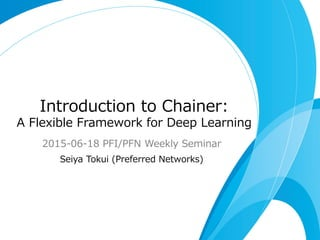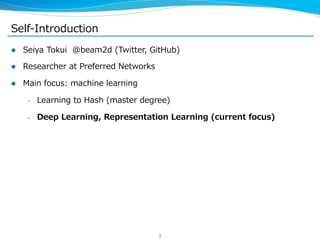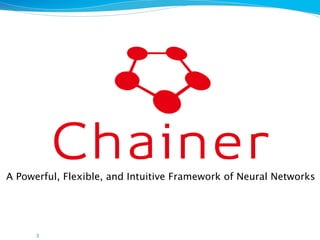Successfully reported this slideshow.

# Introduction to Chainer: A Flexible Framework for Deep Learning

98

Share×
1 of 36
1 of 36

# Introduction to Chainer: A Flexible Framework for Deep Learning

98

Share

This is the slide used for PFI/PFN weekly seminar on June 18, 2015. Video (in Japanese): http://www.ustream.tv/recorded/64082997

This is the slide used for PFI/PFN weekly seminar on June 18, 2015. Video (in Japanese): http://www.ustream.tv/recorded/64082997

## More Related Content

### Related Books

Free with a 14 day trial from Scribd

See all

### Related Audiobooks

Free with a 14 day trial from Scribd

See all

### Introduction to Chainer: A Flexible Framework for Deep Learning

1. 1. Introduction  to  Chainer: A  Flexible  Framework  for  Deep  Learning 2015-‐‑‒06-‐‑‒18  PFI/PFN  Weekly  Seminar Seiya  Tokui  (Preferred  Networks)
2. 2. Self-‐‑‒Introduction l  Seiya  Tokui    @beam2d  (Twitter,  GitHub) l  Researcher  at  Preferred  Networks l  Main  focus:  machine  learning –  Learning  to  Hash  (master  degree) –  Deep  Learning,  Representation  Learning  (current  focus) 2
3. 3. 3 A Powerful, Flexible, and Intuitive Framework of Neural Networks
4. 4. Today  I  will  introduce: l  The  features  of  Chainer l  How  to  use  Chainer l  Some  planned  features l  (Slide  in  English,  talk  in  Japanese)
5. 5. : The Concept 5
6. 6. Chainer  is  a  framework  of  neural  networks l  Oﬃcial  site:  http://chainer.org   l  Repository:  https://github.com/pfnet/chainer l  Provided  as  a  Python  library  (PyPI:  chainer) l  Main  features –  Powerful:Supports  CUDA  and  multi-‐‑‒GPU  capability –  Flexible: Support  almost  arbitrary  architectures –  Intuitive: Forward  prop  can  be  written  as  a  regular  Python  code
7. 7. Elements  of  a  neural  network  framework l  Multi-‐‑‒dimensional  array  implementations l  Layer  implementations –  Called  in  various  names  (layers,  modules,  blocks,  primitives,  etc...) –  The  smallest  units  of  automatic  diﬀerentiation –  Contain  forward  and  backward  implementations l  Optimizer  implementations l  Other  stuﬀs  (data  loading  scheme,  training  loop,  etc...) –  These  are  also  very  important,  though  Chainer  currently  does  not   provide  their  abstraction  (future  work) 7
8. 8. Forward  prop  /  Backprop l  Forward  prop  is  how  we  want  to  process  the  input  data l  Backprop  computes  its  gradient  for  the  learnable  parameters l  Given  backward  procedures  of  all  layers,  backprop  can  be  written  as   their  combination  (a.k.a.  reverse-‐‑‒mode  automatic  diﬀerentiation) 8 input hidden output groundtruth loss  func gradgradgrad hidden
9. 9. Backprop  Implementation  Paradigm  (1) Deﬁne-‐‑‒and-‐‑‒Run l  First,  a  computational  graph  is  constructed.  Then,  it  is  periodically  fed   with  minibatches  to  do  forward/backward l  The  computational  graph  can  be  seen  as  a  program  and  the  forward/ backward  computation  is  done  by  its  interpreter u  Caﬀe:  the  program  is  written  by  Prototxt u  Torch:  the  program  is  constructed  by  Lua  scripts u  Theano-‐‑‒based  frameworks:  the  program  is  constructed  by  Python   scripts
10. 10. Backprop  Implementation  Paradigm  (2) Deﬁne-‐‑‒and-‐‑‒Run  (cont.) l  Pros –  (Almost)  No  need  of  memory  management –  The  computational  graph  can  be  implicitly  optimized  (cf.  Theano) l  Cons –  The  program  is  ﬁxed  within  the  training  loop –  The  interpreter  must  have  capability  of  deﬁning  various  forward   computations,  including  control-‐‑‒ﬂow  statements  like  if  and  for u  Theano  has  the  dedicated  functions  for  them  (ifelse  and  scan),   which  are  unintuitive  and  not  Pythonic –  Network  deﬁnition  is  hard  to  debug,  since  an  error  occurs  at  the   forward  computation  that  is  far  apart  from  the  network  deﬁnition
11. 11. Backprop  Implementation  Paradigm  (3) Deﬁne-‐‑‒by-‐‑‒Run l  The  forward  computation  is  written  as  a  regular  program  code  with   special  variables  and  operators,  executing  which  simultaneously  involves   the  forward  computation  and  the  graph  construction  (just  by  storing  the   order  of  operations). l  The  graph  is  used  for  the  backward  computation. l  This  paradigm  enables  us  to  use  arbitrary  control  ﬂow  statements  in  the   forward  computation –  No  need  of  a  mini  language  and  its  interpreter l  It  also  makes  the  forward  computation  intuitive  and  easy  to  debug
12. 12. Backprop  Implementation  Paradigm  (4) Deﬁne-‐‑‒by-‐‑‒Run  (cont.) l  The  computational  graph  can  be  modiﬁed  within  each  iteration l  Example:  Truncated  BPTT  (BackProp  Through  Time) –  BPTT:  Backprop  on  a  recurrent  net –  Truncated  BPTT:  Truncate  the  backprop  at  some  time  point –  Truncation  is  one  type  of  modiﬁcation  of  the  computational  graph Truncated
13. 13. Features  of  Chainer l  Deﬁne-‐‑‒by-‐‑‒Run  scheme –  Forward  computation  can  contain  any  Python  code u  if-else,  for-else,  break,  continue,  try-except-finally,   list,  dict,  class,  etc... –  User  can  modify  the  graph  within  the  loop u  E.g.  truncation  can  be  done  by  unchain_̲backward  (which   unchains  the  graph  backward  from  some  variable) u  See  the  tutorial  on  recurrent  nets http://docs.chainer.org/en/latest/tutorial/recurrentnet.html l  Predeﬁned  functions l  Support  GPU(s)  via  PyCUDA
14. 14. Example:  Training  a  multi-‐‑‒layer  perceptron  in  one  page Full  code  is  in  the  tutorial  and  the  example  directory. # Model definition model = FunctionSet( l1=F.Linear(784, 100), l2=F.Linear(100, 100), l3=F.Linear(100, 10)) opt = optimizers.SGD() opt.setup( model.collect_parameters()) # Forward computation def forward(x, t): h1 = F.relu(model.l1(x)) h2 = F.relu(model.l2(h1)) y = model.l3(h2) return F.softmax_cross_entropy(y, t) # Training loop for epoch in xrange(n_epoch): for i in xrange(0, N, batchsize): x = Variable(...) t = Variable(...) opt.zero_grads() loss = forward(x, t) loss.backward() opt.update()
15. 15. Example:  Recurrent  net  language  model  in  one  page Full  code  is  in  the  tutorial  and  the  example  directory. # Model definition model = FunctionSet( emb=F.EmbedID(1000, 100), x2h=F.Linear( 100, 50), h2h=F.Linear( 50, 50), h2y=F.Linear( 50, 1000)) opt = optimizers.SGD() opt.setup( model.collect_parameters()) # Forward computation of one step def fwd1step(h, w, t): x = F.tanh(model.emb(w)) h = F.tanh(model.x2h(x) + model.h2h(h)) y = model.h2y(h) return h, F.softmax_cross_entropy(y, t) # Full RNN forward computation def forward(seq): h = Variable(...) # init state loss = 0 for curw, nextw in zip(seq, seq[1:]): x = Variable(curw) t = Variable(nextw) h, new_loss = fwd1step(h, x, t) loss += new_loss return loss
16. 16. : How to Use It 16
17. 17. Install  Chainer l  Prepare  a  Python  2.7  environment  with  pip –  (Pyenv+)Anaconda  is  recommended l  Install  Chainer  just  by pip install chainer l  If  you  want  to  use  GPU(s),  do: –  Install  CUDA  and  the  corresponding  NVIDIA  driver –  Install  dependent  packages  by pip install chainer-cuda-deps –  You  may  have  to  update  the  six package pip install –U six
18. 18. Run  the  MNIST  example  (quick  start) l  Require  scikit-‐‑‒learn  installed:  pip install scikits.learn l  Clone  the  repository  of  Chainer:   git clone https://github.com/pfnet/chainer l  Go  to  the  example  directory  at  examples/mnist l  Then,  run  python train_mnist.py –  Run  on  GPU  by  passing  --gpu=0 l  Other  examples  can  be  similarly  executed  (some  needs  manual   preparation  of  datasets)
19. 19. Read  the  documents l  Read  the  documents  at  http://docs.chainer.org l  It  includes: –  Tutorial –  Reference  manual l  All  features  given  in  this  talk  are  introduced  by  the  tutorial,  so  please  try   it  if  you  want  to  know  the  detail.
20. 20. Basic  concepts  (1) l  Essential  part  of  Chainer:  Variable  and  Function l  Variable  is  a  wrapper  of  n-‐‑‒dimensional  arrays  (ndarray  and  GPUArray) l  Function  is  an  operation  on  Variables –  Function  application  is  memorized  by  the  returned  Variable(s) –  All  operations  for  which  you  want  to  backprop  must  be  done  by   Functions  on  Variables l  Making  a  Variable  object  is  simple:  just  pass  an  array x = chainer.Variable(numpy.ndarray(...)) –  The  array  is  stored  in  data  attribute  (x.data)
21. 21. Basic  concepts  (2) l  Example  of  the  computational  graph  construction x = chainer.Variable(...) y = chainer.Variable(...) z = x**2 + 2*x*y + y l  Gradient  of  z(x,  y)  can  be  computed  by  z.backward() l  Results  are  stored  in  x.grad  and  y.grad x y _ ** 2 2 * _ _ * _ _ + _ z _ + _ Actually, Split nodes are automatically inserted (they accumulate the gradients on backprop)
22. 22. Basic  concepts  (3) l  Chainer  provides  many  functions  in  chainer.functions  subpackage –  This  package  is  often  abbreviated  to  F l  Parameterized  functions  are  provided  as  classes –  Linear,  Convolution2D,  EmbedID,  PReLU,  BatchNormalization,  etc. –  Their  instances  should  be  shared  across  all  iterations l  Non-‐‑‒parameterized  functions  are  provided  as  Python  functions –  Activation  functions,  pooling,  array  manipulation,  etc.
23. 23. Basic  concepts  (4) l  Use  FunctionSet  to  manage  parameterized  functions –  It  is  an  object  with  Function  attributes –  Easy  to  migrate  functions  onto  GPU  devices –  Easy  to  collect  parameters  and  gradients  (collect_̲parameters) l  Use  Optimizer  for  numerical  optimization –  Major  algorithms  are  provided: SGD,  MomentumSGD,  AdaGrad,  RMSprop,  ADADELTA,  Adam –  Some  parameter/gradient  manipulations  are  done  via  this  class: weight  decay,  gradient  clip,
24. 24. Easy  to  debug! l  If  the  forward  computation  has  a  bug,  then  an  error  occurs  immediately   at  the  appropriate  line  of  the  forward  deﬁnition l  Example –  This  code  has  inconsistency  of  the  array  size: x = Variable(np.ndarray((3, 4), dtype=np.float32) y = Variable(np.ndarray((3, 3), dtype=np.float32) a = x ** 2 + x b = a + y * 2 c = b + x * 2 –  Since  an  exception  is  raised  at  the  appropriate  line,  we  can  easily  ﬁnd   the  cause  of  bug  (this  is  one  big  diﬀerence  from  Deﬁne-‐‑‒and-‐‑‒Run   frameworks) ← an exception is raised at this line
25. 25. Graph  manipulation  (1) l  Backward  unchaining:  y.unchain_backward() –  It  purges  the  nodes  backward  from  y –  It  is  useful  to  implement  truncated  BPTT  (see  PTB  example) x f y g z y g z y.unchain_backward()
26. 26. Graph  manipulation  (2) l  Volatile  variables:  x = Variable(..., volatile=True) –  Volatile  variable  does  not  build  a  graph –  Volatility  can  be  accessed  directly  by  x.volatile x = Variable(..., volatile=True) y = f(x) y.volatile = False z = h(y) x f y g z
27. 27. Example:  Training  a  multi-‐‑‒layer  perceptron  in  one  page Note:  F = chainer.functions # Model definition model = FunctionSet( l1=F.Linear(784, 100), l2=F.Linear(100, 100), l3=F.Linear(100, 10)) opt = optimizers.SGD() opt.setup( model.collect_parameters()) # Forward computation def forward(x, t): h1 = F.relu(model.l1(x)) h2 = F.relu(model.l2(h1)) y = model.l3(h2) return F.softmax_cross_entropy(y, t) # Training loop for epoch in xrange(n_epoch): for i in xrange(0, N, batchsize): x = Variable(...) t = Variable(...) opt.zero_grads() loss = forward(x, t) loss.backward() opt.update()
28. 28. Example:  Recurrent  net  language  model  in  one  page # Model definition model = FunctionSet( emb=F.EmbedID(1000, 100), x2h=F.Linear( 100, 50), h2h=F.Linear( 50, 50), h2y=F.Linear( 50, 1000)) opt = optimizers.SGD() opt.setup( model.collect_parameters()) # Forward computation of one step def fwd1step(h, w, t): x = F.tanh(model.emb(w)) h = F.tanh(model.x2h(x) + model.h2h(h)) y = model.h2y(h) return h, F.softmax_cross_entropy(y, t) # Full RNN forward computation def forward(seq): h = Variable(...) # init state loss = 0 for curw, nextw in zip(seq, seq[1:]): x = Variable(curw) t = Variable(nextw) h, new_loss = fwd1step(h, x, t) loss += new_loss return loss
29. 29. CUDA  support  (1) l  Chainer  supports  CUDA  computation l  Installation –  Install  CUDA  6.5+ –  Install  CUDA-‐‑‒related  packages  by pip install chainer-cuda-deps u  Build  of  PyCUDA  may  fail  if  you  install  CUDA  into  non-‐‑‒standard   path.  In  such  case,  you  have  to  install  PyCUDA  from  source  code   with  appropriate  conﬁguration.
30. 30. CUDA  support  (2) l  Call  cuda.init() before  any  CUDA-‐‑‒related  operations l  Converts  numpy.ndarray  into  GPUArray  by  chainer.cuda.to_gpu data_gpu = chainer.cuda.to_gpu(data_cpu) l  A  GPUArray  object  can  be  passed  to  the  Variable  constructor x = Variable(data_gpu) l  Most  functions  support  GPU  Variables –  Parameterized  functions  must  be  sent  to  GPU  beforehand  by   Function.to_gpu  or  FunctionSet.to_gpu l  Extracts  the  results  to  host  memory  by  chainer.cuda.to_cpu l  All  examples  support  CUDA  (pass  --gpu=N,  where  N  is  the  GPU  ID)
31. 31. MLP  example  for  CUDA # Model definition model = FunctionSet( l1=F.Linear(784, 100), l2=F.Linear(100, 100), l3=F.Linear(100, 10)).to_gpu() opt = optimizers.SGD() opt.setup( model.collect_parameters()) # Forward computation def forward(x, t): h1 = F.relu(model.l1(x)) h2 = F.relu(model.l2(h1)) y = model.l3(h2) return F.softmax_cross_entropy(y, t) # Training loop for epoch in xrange(n_epoch): for i in xrange(0, N, batchsize): x = Variable(to_gpu(...)) t = Variable(to_gpu(...)) opt.zero_grads() loss = forward(x, t) loss.backward() opt.update()
32. 32. CUDA  support  (3) l  Chainer  also  supports  computation  on  multiple  GPUs  (easily!) l  Model  parallel –  Send  FunctionSets  to  appropriate  devices  (to_̲gpu  accepts  GPU  ID) model_0 = FunctionSet(...).to_gpu(0) model_1 = FunctionSet(...).to_gpu(1) –  Copy  Variable  objects  across  GPUs  by  copy  function x_1 = F.copy(x_0, 1) u  This  copy  is  tracked  by  the  computational  graph,  so  you  donʼ’t   need  to  deal  with  it  on  backprop
33. 33. CUDA  support  (4) l  Chainer  also  supports  computation  on  multiple  GPUs l  Data  parallel –  FunctionSet  can  be  copied  by  copy.copy model = FunctionSet(...) model_0 = copy.copy(model_0).to_gpu(0) model_1 = model_1.to_gpu(1) –  Set  up  the  optimizer  only  for  the  master  model opt.setup(model_0.collect_parameters()) –  After  data-‐‑‒parallel  gradient  computation,  gather  them opt.accumulate_grads(model_1.gradients) –  After  the  update,  share  them  across  model  copies model_1.copy_parameters_from(model_0.parameters)
34. 34. Model  Zoo  support  (in  the  near  future) l  Model  Zoo  is  a  place  that  pretrained  models  are  registered –  Provided  by  BVLC  Caﬀe  team –  It  contains  the  Caﬀe  reference  models l  We  are  planning  to  support  the  Caﬀe  reference  models  in  three  weeks   (the  next  minor  release) –  Current  design  (it  may  be  changed): f = CaffeFunction(‘path/to/model.caffemodel’) x, t = Variable(...), Variable(...) y = f(inputs={‘data’: x, ‘label’: t}, outputs=[‘loss’]) –  It  emulates  Caﬀe  networks  by  Chainerʼ’s  functions
35. 35. Note:  development  process l  Schedule –  We  are  planning  to  release  updates  biweekly –  Updates  are  classiﬁed  into  three  groups u  Revision:  bug  ﬁxes,  updates  without  adding/modifying  interfaces u  Minor:  Updates  that  add/modify  interfaces  without  lacking   backward  compatibility u  Major:  Updates  that  are  not  backward-‐‑‒compatible l  We  are  using  the  GitHub-‐‑‒ﬂow  process l  We  welcome  your  PRs! –  Please  send  them  to  the  master  branch
36. 36. Wrap  up l  Chainer  is  a  powerful,  ﬂexible,  and  intuitive  framework  of  neural   networks  in  Python l  It  is  based  on  Deﬁne-‐‑‒by-‐‑‒Run  scheme,  which  makes  it  intuitive  and   ﬂexible l  Chainer  is  a  very  young  project  and  immature –  Its  development  started  at  mid.  April  (just  two  months  ago) –  We  will  add  many  functionailities  (especially  more  functions) –  We  may  add  some  abstraction  of  whole  learning  processes